• 机器学习线性回归分析和预测棒球比赛数据。

要成为自己的光呀

文章目录一、查看数据二、线性回归分析与预测

对 baseball_02.csv 里面的数据进行分析，并利用 sklearn 的线性回归模型预测球队的表现
有关MLB的详细信息，请参阅以下中文维基百科页面： https://bk.tw.lvfukeji.com/wiki/MLB 你也可以看电影《金钱球》，了解奥克兰田径队是如何利用分析来重塑棒球队的管理的
分析和代码测试都是在 jupyter notebook 环境中进行的

一、查看数据
# 导入需要用到的包
import numpy as np
import pandas as pd
import seaborn as sns
import matplotlib.pyplot as plt
import matplotlib as mpl
from sklearn import linear_model
%matplotlib inline

# 读取数据  查看前5行数据分析时需要用到的字段的含义

RS：run scored 得分
RA：run allowes 失分
W：win 获胜次数
OBP上垒率/打者不出局率：安打数+保送数+触身球数/打数+保送数+触身球数
SLG长打率/衡量打者长打能力：一垒打数+二垒打数2+三垒打数3+全垒打数*4/该打者的打数
BA打击率：安打数/打数
Playoffs 季后赛：0：未进入季后赛    1：进入季后赛
OOBP：对手的基本百分比
OSLG：对手的重击百分比

二、线性回归分析与预测

第一个预测问题是
一支球队要在常规赛中赢多少场才能进入季后赛？
现在，你可以将常规赛简化成一道数学题，利用1995年至2001年的数据绘制散点图。

# 分组  进入季后赛 与 未进入季后赛分组 统计各自最小的胜投次数
baseball.groupby('Playoffs').min()['W']从历史数据来看，要进入季后赛，至少需要赢 82 场
# -*- coding: UTF-8 -*-
"""
@Author  ：叶庭云
@公众号  ：修炼Python
@CSDN    ：https://yetingyun.blog.csdn.net/
"""
# 条件筛选 从1995年至2001年且进入季后赛的队伍  统计这些队伍的获胜次数  散点图可视化
baseball2 = baseball[baseball["Year"]>=1995].copy()
baseball2 = baseball2[baseball2["Year"]<=2001].copy()
baseball2 = baseball2[baseball2["Playoffs"]==1].copy()
plt.figure(figsize=(12, 8), dpi=120)
mpl.rcParams['font.family'] = 'SimHei'
plt.style.use('ggplot')
plt.scatter(range(baseball2.shape),baseball2["W"],color='red')
plt.xlabel('各个队伍')
plt.ylabel('胜投数')
plt.savefig('test_01.png')
baseball2.shape     # (48, 15)从散点图容易看出，有一个最小值82，但大部分散点在 85 以上，所以一支球队要在常规赛中获胜 85 场以上，进入季后赛的概率很大。

思考一个团队如何才能取得 X 场胜利
当一个队的得分比对手多时，它就会获胜。但是，球队要赢了多少次？
使用一个线性回归模型，回答一个问题：如何能使一个球队获胜，在常规赛中，它的得分需要比失分多多少分？
首先，您只使用2002年以前的数据

baseball2 = baseball[baseball["Year"]<=2002].copy()


为了使问题更简单，您可以创建一个变量来保存球队得分和球队失分的差。
然后，您可以将该变量用作线性回归模型中的单个自变量，因变量是获胜次数。

# -*- coding: UTF-8 -*-
"""
@Author  ：叶庭云
@公众号  ：修炼Python
@CSDN    ：https://yetingyun.blog.csdn.net/
"""
baseball2["newVar"] = baseball2["RS"] - baseball2["RA"]
reg1 = linear_model.LinearRegression()
# 球队得分和球队失分的差
x = baseball2["newVar"].values.reshape(-1,1)
# 获胜次数
y = baseball2["W"].values.reshape(-1,1)
reg1.fit(x,y)
yPred = reg1.predict(x)
# 预测至少胜 85 场进季后赛  在常规赛中，球队的得分需要比失分多89.858009分
predict_sample = reg1.predict([])
print(predict_sample)

plt.figure(figsize=(12, 8), dpi=120)
plt.rcParams['axes.unicode_minus']=False  # 用于解决不能显示负号的问题
plt.clf()
# fig,ax = plt.subplots(1,1)
plt.scatter(x,y,label="true",color='blue')
plt.scatter(x,yPred,label="pred",color='red')
plt.xlabel('得分与失分之差')
plt.ylabel('获胜次数')
plt.savefig('test_02.png')   # 保存图片
plt.legend()   # 显示图例根据拟合的线性回归模型，如果一支球队想要赢得 85 场胜利，它球队的得分比失分需要多大约 90 分。

现在，从上面的线性回归模型中，你知道一个团队应该比它允许的 X 胜是多少次。
从本质上讲，一支球队的得分应该超过它允许的获胜次数。接下来，我们要预测球队的得分和允许的失分。
关于得分，假设两个棒球统计数据比其他任何东西都重要：
（1）上垒百分比（OBP）：玩家在垒上的时间百分比（包括步行）
（2）重击百分比（SLG）：玩家在回合中绕垒的距离（测量力量）
（3）对于你的回归模型，你还包括另一个变量，（BA）：击球得分

# 运行一个带有上述三个变量的线性回归模型来预测得分
x = baseball2[["OBP","SLG","BA"]].values.reshape(-1,3)
y = baseball2[["RS"]].values.reshape(-1,1)
reg2 = linear_model.LinearRegression()
reg2.fit(x,y)
yPred = reg2.predict(x)
baseball2["3var_pred"] = yPred# 运行另一个只有两个变量的线性回归模型，OBP和SLG
x = baseball2[["OBP","SLG"]].values.reshape(-1,2)
y = baseball2[["RS"]].values.reshape(-1,1)
reg3 = linear_model.LinearRegression()
reg3.fit(x,y)
yPred = reg3.predict(x)
baseball2["OBP SLG pred"] = yPred如果一支棒球队的OBP为0.311，SLG为0.405，我们期望该队得分多少分？

x = np.array([0.311,0.405]).reshape(-1,2)
var2_Pred = reg3.predict(x)
print(var2_Pred)我们可以使用线性回归模型来预测允许的失分。
使用以下两个变量，OOBP（对手的基本百分比）和OSLG（对手的重击百分比）。

print(baseball.columns)
baseball3 = baseball.dropna(subset=['OOBP'], axis=0, inplace=False)
baseball3 = baseball.dropna(subset=['OSLG'], axis=0, inplace=False)
x = baseball3[["OOBP","OSLG"]].values.reshape(-1,2)
y = baseball3[["RA"]].values.reshape(-1,1)
reg4 = linear_model.LinearRegression()
reg4.fit(x,y)
yPred = reg4.predict(x)
baseball3["OOBP OSLG pred"] = yPred如果一支棒球队的对手OBP（OOBP）为0.297，对手SLG（OSLG）为0.370，预期球队允许失分多少？

x = np.array([0.297,0.370]).reshape(-1,2)
yPred = reg4.predict(x)
print(yPred)现在让我们假设我们使用线性回归模型来预测2002年奥克兰A队将赢得多少场比赛
在我们的数据中，‘Team’ 变量中的 OAK 代表oaklanda。
运行的模型使用团队统计数据。我们将使用 2001 年的团队统计数据来预测 2002 年的情况。
2001年奥克兰A队的 OBP 和 SLG 是多少？根据我们的得分模型，这个队预计能得分多少？

# 提取2001年oaklanda的数据
baseball2 = baseball[baseball["Team"]=="OAK"].copy()
baseball2 = baseball2[baseball2["Year"]==2001].copy()[["OBP","SLG"]]
x = baseball2.values.reshape(-1,2)
yPred = reg4.predict(x)
print()
print(yPred)   # 预测得分
RS = yPred2001年奥克兰A队的OOBP和OSLG是多少？根据我们的允许失分模型，该球队预计失分多少

baseball2 = baseball[baseball["Team"]=="OAK"].copy()
baseball2 = baseball2[baseball2["Year"]==2001].copy()[["OOBP","OSLG"]]
x = baseball2.values.reshape(-1,2)
yPred = reg3.predict(x)
print()
print(yPred)    # 预测失分
RA = yPred现在我们已经预测了2002年奥克兰A的得分和失分。根据预测和我们的获胜模型，预计球队会赢多少场？

x = np.array(RS - RA).reshape(-1,1)
wPred = reg1.predict(x)
print(wPred)
# 大约会赢101场根据预测的获胜次数，你预测球队是否会在2002年进入季后赛？

这支球队会在2002年进入季后赛，根据线性回归模型预测出的获胜的场数约为101场，大于85。

从我们的数据中，你可以了解2002年奥克兰A队的实际表现。
你认为你的预测在得分、允许跑数和获胜数方面是否接近实际表现？

# -*- coding: UTF-8 -*-
"""
@Author  ：叶庭云
@公众号  ：修炼Python
@CSDN    ：https://yetingyun.blog.csdn.net/
"""
oak2002 = baseball[baseball["Team"]=="OAK"].copy()
oak2002 = oak2002[oak2002["Year"]==2002].copy()
print(f'Playoffs：{oak2002["Playoffs"].values}')
print("事实  RS:%.2f  RA:%.2f  W:%.2f"%(oak2002["RS"],oak2002["RA"],oak2002["W"]))
print("预测  RS:%.2f  RA:%.2f  W:%.2f"%(RS,RA,wPred))这支球队 2002 年的确进入季后赛了，预测的得分、失分、获胜场数与真实数据相比差异不大，因为每年团队层面的差异并不大
所以用 2001 年的数据来预测 2002 年的数据也不会有太大的差异，误差较小。


展开全文python
• 本文介绍回归模型的原理知识，包括线性回归、多项式回归和逻辑回归，并详细介绍Python Sklearn机器学习库的LinearRegression和LogisticRegression算法及回归分析实例。进入基础文章，希望对您有所帮助。
欢迎大家来到“Python从零到壹”，在这里我将分享约200篇Python系列文章，带大家一起去学习和玩耍，看看Python这个有趣的世界。所有文章都将结合案例、代码和作者的经验讲解，真心想把自己近十年的编程经验分享给大家，希望对您有所帮助，文章中不足之处也请海涵。Python系列整体框架包括基础语法10篇、网络爬虫30篇、可视化分析10篇、机器学习20篇、大数据分析20篇、图像识别30篇、人工智能40篇、Python安全20篇、其他技巧10篇。您的关注、点赞和转发就是对秀璋最大的支持，知识无价人有情，希望我们都能在人生路上开心快乐、共同成长。
前一篇文章讲述了数据分析部分，主要普及网络数据分析的基本概念，讲述数据分析流程和相关技术，同时详细讲解Python提供的若干第三方数据分析库，包括Numpy、Pandas、Matplotlib、Sklearn等。本文介绍回归模型的原理知识，包括线性回归、多项式回归和逻辑回归，并详细介绍Python Sklearn机器学习库的LinearRegression和LogisticRegression算法及回归分析实例。进入基础文章，希望对您有所帮助。
文章目录一.回归1.什么是回归2.线性回归二.线性回归分析1.LinearRegression2.线性回归预测糖尿病三.多项式回归分析1.基础概念2.PolynomialFeatures3.多项式回归预测成本和利润四.逻辑回归1.基础原理2.LogisticRegression3.鸢尾花数据集回归分析实例五.本章小结
下载地址：

https://github.com/eastmountyxz/Python-zero2one

前文赏析：
第一部分 基础语法

[Python从零到壹] 一.为什么我们要学Python及基础语法详解
[Python从零到壹] 二.语法基础之条件语句、循环语句和函数
[Python从零到壹] 三.语法基础之文件操作、CSV文件读写及面向对象

第二部分 网络爬虫

[Python从零到壹] 四.网络爬虫之入门基础及正则表达式抓取博客案例
[Python从零到壹] 五.网络爬虫之BeautifulSoup基础语法万字详解
[Python从零到壹] 六.网络爬虫之BeautifulSoup爬取豆瓣TOP250电影详解
[Python从零到壹] 七.网络爬虫之Requests爬取豆瓣电影TOP250及CSV存储
[Python从零到壹] 八.数据库之MySQL基础知识及操作万字详解
[Python从零到壹] 九.网络爬虫之Selenium基础技术万字详解（定位元素、常用方法、键盘鼠标操作）
[Python从零到壹] 十.网络爬虫之Selenium爬取在线百科知识万字详解（NLP语料构造必备技能）

第三部分 数据分析和机器学习

[Python从零到壹] 十一.数据分析之Numpy、Pandas、Matplotlib和Sklearn入门知识万字详解(1)
[Python从零到壹] 十二.机器学习之回归分析万字总结全网首发（线性回归、多项式回归、逻辑回归）

作者新开的“娜璋AI安全之家”将专注于Python和安全技术，主要分享Web渗透、系统安全、人工智能、大数据分析、图像识别、恶意代码检测、CVE复现、威胁情报分析等文章。虽然作者是一名技术小白，但会保证每一篇文章都会很用心地撰写，希望这些基础性文章对你有所帮助，在Python和安全路上与大家一起进步。

监督学习（Supervised Learning）包括分类算法(Classification)和回归算法（Regression）两种，它们是根据类别标签分布的类型来定义的。回归算法用于连续型的数据预测，分类算法用于离散型的分布预测。回归算法作为统计学中最重要的工具之一，它通过建立一个回归方程用来预测目标值，并求解这个回归方程的回归系数。
一.回归
1.什么是回归
回归（Regression）最早是英国生物统计学家高尔顿和他的学生皮尔逊在研究父母和子女的身高遗传特性时提出的。1855年，他们在《遗传的身高向平均数方向的回归》中这样描述“子女的身高趋向于高于父母的身高的平均值，但一般不会超过父母的身高”，首次提出来回归的概念。现在的回归分析已经和这种趋势效应没有任何瓜葛了，它只是指源于高尔顿工作，用一个或多个自变量来预测因变量的数学方法。图1是一个简单的回归模型，X坐标是质量，Y坐标是用户满意度，从图中可知，产品的质量越高其用户评价越好，这可以拟合一条直线来预测新产品的用户满意度。
在回归模型中，我们需要预测的变量叫做因变量，比如产品质量；选取用来解释因变量变化的变量叫做自变量，比如用户满意度。回归的目的就是建立一个回归方程来预测目标值，整个回归的求解过程就是求这个回归方程的回归系数。
简言之，回归最简单的定义就是：

给出一个点集，构造一个函数来拟合这个点集，并且尽可能的让该点集与拟合函数间的误差最小，如果这个函数曲线是一条直线，那就被称为线性回归，如果曲线是一条三次曲线，就被称为三次多项回归。

2.线性回归
首先，作者引用类似于斯坦福大学机器学习公开课线性回归的例子，给大家讲解线性回归的基础知识和应用，方便大家的理解。同时，作者强烈推荐大家学习原版Andrew Ng教授的斯坦福机器学习公开课，会让您非常受益。假设存在表1的数据集，它是某企业的成本和利润数据集。数据集中2002年到2016年的数据集称为训练集，整个训练集共15个样本数据。重点是成本和利润两个变量，成本是输入变量或一个特征，利润是输出变量或目标变量，整个回归模型如图2所示。现建立模型，x表示企业成本，y表示企业利润，h（Hypothesis）表示将输入变量映射到输出变量y的函数，对应一个因变量的线性回归（单变量线性回归）公式如下：那么，现在要解决的问题是如何求解的两个参数和。我们的构想是选取的参数和使得函数尽可能接近y值，这里提出了求训练集(x,y)的平方误差函数（Squared Error Function）或最小二乘法。
在回归方程里，最小化误差平方和方法是求特征对应回归系数的最佳方法。误差是指预测y值和真实y值之间的差值，使用误差的简单累加将使得正差值和负差值相互抵消，所采用的平方误差（最小二乘法）如下：选择适当的参数让其最小化min，即可实现拟合求解过程。通过上面的这个示例，我们就可以对线性回归模型进行如下定义：根据样本x和y的坐标，去预估函数h，寻求变量之间近似的函数关系。公式如下：其中，n表示特征数目，表示每个训练样本的第i个特种值，当只有一个因变量x时，称为一元线性回归，类似于；而当多个因变量时，成为多元线性回归。我们的目的是使最小化，从而最好的将样本数据集进行拟合，更好地预测新的数据。

多项式回归或逻辑回归相关知识将在后面介绍。

二.线性回归分析
线性回归是数据挖掘中基础的算法之一，其核心思想是求解一组因变量和自变量之间的方程，得到回归函数，同时误差项通常使用最小二乘法进行计算。在本书常用的Sklaern机器学习包中将调用Linear_model子类的LinearRegression类进行线性回归模型计算。
1.LinearRegression
LinearRegression回归模型在Sklearn.linear_model子类下，主要是调用fit(x,y)函数来训练模型，其中x为数据的属性，y为所属类型。sklearn中引用回归模型的代码如下：
from sklearn import linear_model          #导入线性模型
regr = linear_model.LinearRegression()    #使用线性回归
print(regr)

输出函数的构造方法如下：
LinearRegression(copy_X=True,
fit_intercept=True,
n_jobs=1,
normalize=False)

其中参数说明如下：

copy_X：布尔型，默认为True。是否对X复制，如果选择False，则直接对原始数据进行覆盖，即经过中心化、标准化后，把新数据覆盖到原数据上。
fit_intercept：布尔型，默认为True。是否对训练数据进行中心化，如果是True表示对输入的训练数据进行中心化处理，如果是False则输入数据已经中心化处理，后面的过程不再进行中心化处理。
n_jobs：整型，默认为1。计算时设置的任务个数，如果设置为-1表示使用所有的CPU。该参数对于目标个数大于1且规模足够大的问题有加速作用。
normalize：布尔型，默认为False。是否对数据进行标准化处理。

LinearRegression类主要包括如下方法：fit(X,y[,n_jobs])
对训练集X，y进行训练，分析模型参数，填充数据集。其中X为特征，y为标记或类属性。
predict(X)
使用训练得到的估计器或模型对输入的X数据集进行预测，返回结果为预测值。数据集X通常划分为训练集和测试集。
decision_function(X)
使用训练得到的估计器或模型对数据集X进行预测。它与predict(X)区别在于该方法包含了对输入数据的类型检查和当前对象是否存在coef_属性的检查，更安全。
score(X, y[,]samples_weight)
返回对于以X为samples、y为target的预测效果评分。
get_params([deep])
获取该估计器（Estimator）的参数。
**set_params(params)
设置该估计器（Estimator）的参数。
coef_
存放LinearRegression模型的回归系数。
intercept_
存放LinearRegression模型的回归截距。

现在对前面的企业成本和利润数据集进行线性回归实验。完整代码如下：
# -*- coding: utf-8 -*-
# By:Eastmount CSDN 2021-07-03
from sklearn import linear_model     #导入线性模型
import matplotlib.pyplot as plt
import numpy as np

#X表示企业成本 Y表示企业利润
X = [, , , , , , , , , , , , , , ]
Y = [, , , , , , , , , , , , , , ]
print('数据集X: ', X)
print('数据集Y: ', Y)

#回归训练
clf = linear_model.LinearRegression()
clf.fit(X, Y)

#预测结果
X2 = [, , ]
Y2 = clf.predict(X2)
print(Y2)
res = clf.predict(np.array().reshape(-1, 1))
print('预测成本1200元的利润：$%.1f' % res) #绘制线性回归图形 plt.plot(X, Y, 'ks') #绘制训练数据集散点图 plt.plot(X2, Y2, 'g-') #绘制预测数据集直线 plt.show()  调用sklearn包中的LinearRegression()回归函数，fit(X,Y)载入数据集进行训练，然后通过predict(X2)预测数据集X2的利润，并将预测结果绘制成直线，(X,Y)数据集绘制成散点图，如图3所示。同时调用代码预测2017年企业成本为1200元的利润为575.1元。注意，线性模型的回归系数会保存在coef_变量中，截距保存在intercept_变量中。clf.score(X, Y) 是一个评分函数，返回一个小于1的得分。评分过程的代码如下： print('系数', clf.coef_) print('截距', clf.intercept_) print('评分函数', clf.score(X, Y)) ''' 系数 [[ 0.62402912]] 截距 [-173.70433885] 评分函数 0.911831188777 '''该直线对应的回归函数为：y = 0.62402912 * x - 173.70433885，则X2=400这个点预测的利润值为75.9，而X1中成本为400元对应的真实利润是80元，预测是基本准确的。 2.线性回归预测糖尿病 (1).糖尿病数据集 Sklearn机器学习包提供了糖尿病数据集（Diabetes Dataset），该数据集主要包括442行数据，10个特征值，分别是：年龄（Age）、性别（Sex）、体质指数（Body mass index）、平均血压（Average Blood Pressure）、S1~S6一年后疾病级数指标。预测指标为Target，它表示一年后患疾病的定量指标。原网址的描述如图4所示：下面代码进行简单的调用及数据规模的测试。 # -*- coding: utf-8 -*- # By:Eastmount CSDN 2021-07-03 from sklearn import datasets diabetes = datasets.load_diabetes() #载入数据 print(diabetes.data) #数据 print(diabetes.target) #类标 print('总行数: ', len(diabetes.data), len(diabetes.target)) print('特征数: ', len(diabetes.data)) #每行数据集维数 print('数据类型: ', diabetes.data.shape) print(type(diabetes.data), type(diabetes.target))  调用load_diabetes()函数载入糖尿病数据集，然后输出其数据data和类标target。输出总行数442行，特征数共10个，类型为（442L, 10L）。其输出如下所示： [[ 0.03807591 0.05068012 0.06169621 ..., -0.00259226 0.01990842 -0.01764613] [-0.00188202 -0.04464164 -0.05147406 ..., -0.03949338 -0.06832974 -0.09220405] ... [-0.04547248 -0.04464164 -0.0730303 ..., -0.03949338 -0.00421986 0.00306441]] [ 151. 75. 141. 206. 135. 97. 138. 63. 110. 310. 101. ... 64. 48. 178. 104. 132. 220. 57.] 总行数: 442 442 特征数: 10 数据类型: (442L, 10L) <type 'numpy.ndarray'> <type 'numpy.ndarray'>  (2).代码实现 现在我们将糖尿病数据集划分为训练集和测试集，整个数据集共442行，我们取前422行数据用来线性回归模型训练，后20行数据用来预测。其中取预测数据的代码为diabetes_x_temp[-20:]，表示从后20行开始取值，直到数组结束，共取值20个数。 整个数据集共10个特征值，为了方便可视化画图我们只获取其中一个特征进行实验，这也可以绘制图形，而真实分析中，通常经过降维处理再绘制图形。这里获取第3个特征，对应代码为：diabetes_x_temp = diabetes.data[:, np.newaxis, 2]。完整代码如下： # -*- coding: utf-8 -*- # By:Eastmount CSDN 2021-07-03 from sklearn import datasets import matplotlib.pyplot as plt from sklearn import linear_model import numpy as np #数据集划分 diabetes = datasets.load_diabetes() #载入数据 diabetes_x_temp = diabetes.data[:, np.newaxis, 2] #获取其中一个特征 diabetes_x_train = diabetes_x_temp[:-20] #训练样本 diabetes_x_test = diabetes_x_temp[-20:] #测试样本 后20行 diabetes_y_train = diabetes.target[:-20] #训练标记 diabetes_y_test = diabetes.target[-20:] #预测对比标记 #回归训练及预测 clf = linear_model.LinearRegression() clf.fit(diabetes_x_train, diabetes_y_train) #训练数据集 pre = clf.predict(diabetes_x_test) #绘图 plt.title(u'LinearRegression Diabetes') #标题 plt.xlabel(u'Attributes') #x轴坐标 plt.ylabel(u'Measure of disease') #y轴坐标 plt.scatter(diabetes_x_test, diabetes_y_test, color = 'black') #散点图 plt.plot(diabetes_x_test, pre, color='blue', linewidth = 2) #预测直线 plt.show()  输出结果如图5所示，每个点表示真实的值，而直线表示预测的结果。(3).代码优化 下面代码增加了几个优化措施，包括增加了斜率、 截距的计算，可视化绘图增加了散点到线性方程的距离线，增加了保存图片设置像素代码等。这些优化都更好地帮助我们分析真实的数据集。 # -*- coding: utf-8 -*- # By:Eastmount CSDN 2021-07-03 from sklearn import datasets import numpy as np from sklearn import linear_model import matplotlib.pyplot as plt #第一步 数据集划分 d = datasets.load_diabetes() #数据 10*442 x = d.data x_one = x[:,np.newaxis, 2] #获取一个特征 第3列数据 y = d.target #获取的正确结果 x_train = x_one[:-42] #训练集X [ 0:400] x_test = x_one[-42:] #预测集X [401:442] y_train = y[:-42] #训练集Y [ 0:400] y_test = y[-42:] #预测集Y [401:442] #第二步 线性回归实现 clf = linear_model.LinearRegression() print(clf) clf.fit(x_train, y_train) pre = clf.predict(x_test) print('预测结果', pre) print('真实结果', y_test) #第三步 评价结果 cost = np.mean(y_test-pre)**2 #2次方 print('平方和计算:', cost) print('系数', clf.coef_) print('截距', clf.intercept_) print('方差', clf.score(x_test, y_test)) #第四步 绘图 plt.plot(x_test, y_test, 'k.') #散点图 plt.plot(x_test, pre, 'g-') #预测回归直线 #绘制点到直线距离 for idx, m in enumerate(x_test): plt.plot([m, m],[y_test[idx], pre[idx]], 'r-') plt.savefig('blog12-01.png', dpi=300) #保存图片 plt.show()  绘制的图形如图6所示。输出结果如下： LinearRegression(copy_X=True, fit_intercept=True, n_jobs=1, normalize=False) 预测结果 [ 196.51241167 109.98667708 121.31742804 245.95568858 204.75295782 270.67732703 75.99442421 241.8354155 104.83633574 141.91879342 126.46776938 208.8732309 234.62493762 152.21947611 159.42995399 161.49009053 229.47459628 221.23405012 129.55797419 100.71606266 118.22722323 168.70056841 227.41445974 115.13701842 163.55022706 114.10695016 120.28735977 158.39988572 237.71514243 121.31742804 98.65592612 123.37756458 205.78302609 95.56572131 154.27961264 130.58804246 82.17483382 171.79077322 137.79852034 137.79852034 190.33200206 83.20490209] 真实结果 [ 175. 93. 168. 275. 293. 281. 72. 140. 189. 181. 209. 136. 261. 113. 131. 174. 257. 55. 84. 42. 146. 212. 233. 91. 111. 152. 120. 67. 310. 94. 183. 66. 173. 72. 49. 64. 48. 178. 104. 132. 220. 57.] 平方和计算: 83.192340827 系数 [ 955.70303385] 截距 153.000183957 方差 0.427204267067  其中cost = np.mean(y_test-pre)**2表示计算预测结果和真实结果之间的平方和，为83.192340827，根据系数和截距得出其方程为：y = 955.70303385 * x + 153.000183957。 三.多项式回归分析 1.基础概念 线性回归研究的是一个目标变量和一个自变量之间的回归问题，但有时候在很多实际问题中，影响目标变量的自变量往往不止一个，而是多个，比如绵羊的产毛量这一变量同时受到绵羊体重、胸围、体长等多个变量的影响，因此需要设计一个目标变量与多个自变量间的回归分析，即多元回归分析。由于线性回归并不适用于所有的数据，我们需要建立曲线来适应我们的数据，现实世界中的曲线关系很多都是增加多项式实现的，比如一个二次函数模型：再或者一个三次函数模型：这两个模型我们绘制的图形如下所示：多项式回归（Polynomial Regression）是研究一个因变量与一个或多个自变量间多项式的回归分析方法。如果自变量只有一个时，称为一元多项式回归；如果自变量有多个时，称为多元多项式回归。在一元回归分析中，如果依变量y与自变量x的关系为非线性的，但是又找不到适当的函数曲线来拟合，则可以采用一元多项式回归。17.3小节主要讲解一元多次的多项式回归分析，一元m次多项式方程如下：其方程的求解过程希望读者下来自行学习，接下来作者主要讲解Python如何代码实现多项式回归分析的。 2.PolynomialFeatures Python的多项式回归需要导入sklearn.preprocessing子类中PolynomialFeatures类实现。PolynomialFeatures对应的函数原型如下： class sklearn.preprocessing.PolynomialFeatures(degree=2, interaction_only=False, include_bias=True)  PolynomialFeatures类在Sklearn官网给出的解释是：专门产生多项式的模型或类，并且多项式包含的是相互影响的特征集。共有三个参数，degree表示多项式阶数，一般默认值是2；interaction_only如果值是true（默认是False），则会产生相互影响的特征集；include_bias表示是否包含偏差列。 PolynomialFeatures类通过实例化一个多项式，建立等差数列矩阵，然后进行训练和预测，最后绘制相关图形，接下来与前面的一元线性回归分析进行对比试验。 3.多项式回归预测成本和利润 本小节主要讲解多项式回归分析实例，分析的数据集是表17.1提供的企业成本和利润数据集。下面直接给出线性回归和多项式回归分析对比的完整代码和详细注释。 # -*- coding: utf-8 -*- # By:Eastmount CSDN 2021-07-03 from sklearn.linear_model import LinearRegression from sklearn.preprocessing import PolynomialFeatures import matplotlib.pyplot as plt import numpy as np #X表示企业成本 Y表示企业利润 X = [, , , , , , , , , , , , , , ] Y = [, , , , , , , , , , , , , , ] print('数据集X: ', X) print('数据集Y: ', Y) #第一步 线性回归分析 clf = LinearRegression() clf.fit(X, Y) X2 = [, , ] Y2 = clf.predict(X2) print(Y2) res = clf.predict(np.array().reshape(-1, 1)) print('预测成本1200元的利润：$%.1f' % res)
plt.plot(X, Y, 'ks')    #绘制训练数据集散点图
plt.plot(X2, Y2, 'g-')  #绘制预测数据集直线

#第二步 多项式回归分析
xx = np.linspace(350,950,100) #350到950等差数列
quadratic_featurizer = PolynomialFeatures(degree = 2) #实例化一个二次多项式

#把训练好X值的多项式特征实例应用到一系列点上,形成矩阵
label="$y = ax^2 + bx + c$",linewidth=2)
plt.legend()
plt.show()

输出图形如下图所示，其中黑色散点图表示真实的企业成本和利润的关系，绿色直线为一元线性回归方程，红色虚曲线为二次多项式方程。它更接近真实的散点图。这里我们使用R方（R-Squared）来评估多项式回归预测的效果，R方也叫确定系数（Coefficient of Determination），它表示模型对现实数据拟合的程度。计算R方的方法有几种，一元线性回归中R方等于皮尔逊积矩相关系数（Pearson Product Moment Correlation Coefficient）的平方，该方法计算的R方是一定介于0～1之间的正数。另一种是Sklearn库提供的方法来计算R方。R方计算代码如下：
print('1 r-squared', clf.score(X, Y))

输出如下所示：
('1 r-squared', 0.9118311887769025)
('2 r-squared', 0.94073599498559335)一元线性回归的R方值为0.9118，多项式回归的R方值为0.9407，说明数据集中超过94%的价格都可以通过模型解释。最后补充5次项的拟合过程，下面只给出核心代码。
# -*- coding: utf-8 -*-
# By:Eastmount CSDN 2021-07-03
from sklearn.linear_model import LinearRegression
from sklearn.preprocessing import PolynomialFeatures
import matplotlib.pyplot as plt
import numpy as np

#X表示企业成本 Y表示企业利润
X = [, , , , , , , , , , , , , , ]
Y = [, , , , , , , , , , , , , , ]
print('数据集X: ', X)
print('数据集Y: ', Y)

#第一步 线性回归分析
clf = LinearRegression()
clf.fit(X, Y)
X2 = [, , ]
Y2 = clf.predict(X2)
print(Y2)
res = clf.predict(np.array().reshape(-1, 1))
print('预测成本1200元的利润：$%.1f' % res) plt.plot(X, Y, 'ks') #绘制训练数据集散点图 plt.plot(X2, Y2, 'g-') #绘制预测数据集直线 #第二步 多项式回归分析 xx = np.linspace(350,950,100) quadratic_featurizer = PolynomialFeatures(degree = 5) x_train_quadratic = quadratic_featurizer.fit_transform(X) X_test_quadratic = quadratic_featurizer.transform(X2) regressor_quadratic = LinearRegression() regressor_quadratic.fit(x_train_quadratic, Y) #把训练好X值的多项式特征实例应用到一系列点上,形成矩阵 xx_quadratic = quadratic_featurizer.transform(xx.reshape(xx.shape, 1)) plt.plot(xx, regressor_quadratic.predict(xx_quadratic), "r--", label="$y = ax^2 + bx + c\$",linewidth=2)
plt.legend()
plt.show()
print('1 r-squared', clf.score(X, Y))

# ('1 r-squared', 0.9118311887769025)
# ('5 r-squared', 0.98087802460869788)

输出如下所示，其中红色虚线为五次多项式曲线，它更加接近真实数据集的分布情况，而绿色直线为一元线性回归方程，显然相较于五次多项式曲线，线性方程拟合的结果更差。同时，五次多项式曲线的R方值为98.08%，非常准确的预测了数据趋势。最后补充一点，建议多项式回归的阶数不要太高，否则会出现过拟合现象。

四.逻辑回归
1.基础原理
在前面讲述的回归模型中，处理的因变量都是数值型区间变量，建立的模型描述是因变量的期望与自变量之间的线性关系或多项式曲线关系。比如常见的线性回归模型：而在采用回归模型分析实际问题中，所研究的变量往往不全是区间变量而是顺序变量或属性变量，比如二项分布问题。通过分析年龄、性别、体质指数、平均血压、疾病指数等指标，判断一个人是否换糖尿病，Y=0表示未患病，Y=1表示患病，这里的响应变量是一个两点（0或1）分布变量，它就不能用h函数连续的值来预测因变量Y（Y只能取0或1）。
总之，线性回归或多项式回归模型通常是处理因变量为连续变量的问题，如果因变量是定性变量，线性回归模型就不再适用了，此时需采用逻辑回归模型解决。
逻辑回归（Logistic Regression）是用于处理因变量为分类变量的回归问题，常见的是二分类或二项分布问题，也可以处理多分类问题，它实际上是属于一种分类方法。二分类问题的概率与自变量之间的关系图形往往是一个S型曲线，如图17.10所示，采用的Sigmoid函数实现。这里我们将该函数定义如下：函数的定义域为全体实数，值域在[0,1]之间，x轴在0点对应的结果为0.5。当x取值足够大的时候，可以看成0或1两类问题，大于0.5可以认为是1类问题，反之是0类问题，而刚好是0.5，则可以划分至0类或1类。对于0-1型变量，y=1的概率分布公式定义如下：y=0的概率分布公式定义如下：其离散型随机变量期望值公式如下：采用线性模型进行分析，其公式变换如下：而实际应用中，概率p与因变量往往是非线性的，为了解决该类问题，我们引入了logit变换，使得logit§与自变量之间存在线性相关的关系，逻辑回归模型定义如下：通过推导，概率p变换如下，这与Sigmoid函数相符，也体现了概率p与因变量之间的非线性关系。以0.5为界限，预测p大于0.5时，我们判断此时y更可能为1，否则y为0。得到所需的Sigmoid函数后，接下来只需要和前面的线性回归一样，拟合出该式中n个参数θ即可。下列为绘制Sigmoid曲线，输出如图10所示。
# -*- coding: utf-8 -*-
# By:Eastmount CSDN 2021-07-03
import matplotlib.pyplot as plt
import numpy as np

def Sigmoid(x):
return 1.0 / (1.0 + np.exp(-x))

x= np.arange(-10, 10, 0.1)
h = Sigmoid(x)                #Sigmoid函数
plt.plot(x, h)
plt.axvline(0.0, color='k')   #坐标轴上加一条竖直的线（0位置）
plt.axhspan(0.0, 1.0, facecolor='1.0', alpha=1.0, ls='dotted')
plt.axhline(y=0.5, ls='dotted', color='k')
plt.yticks([0.0, 0.5, 1.0])  #y轴标度
plt.ylim(-0.1, 1.1)          #y轴范围
plt.show()

由于篇幅有限，逻辑回归构造损失函数J函数，求解最小J函数及回归参数θ的方法就不在叙述，原理和前面介绍的一样，请读者下去深入研究。2.LogisticRegression
LogisticRegression回归模型在Sklearn.linear_model子类下，调用sklearn逻辑回归算法步骤比较简单，即：

导入模型。调用逻辑回归LogisticRegression()函数。
fit()训练。调用fit(x,y)的方法来训练模型，其中x为数据的属性，y为所属类型。
predict()预测。利用训练得到的模型对数据集进行预测，返回预测结果。

代码如下：
# -*- coding: utf-8 -*-
# By:Eastmount CSDN 2021-07-03
from sklearn.linear_model import LogisticRegression  #导入逻辑回归模型
clf = LogisticRegression()
print(clf)
clf.fit(train_feature,label)
predict['label'] = clf.predict(predict_feature)

输出函数的构造方法如下：
LogisticRegression(C=1.0, class_weight=None, dual=False, fit_intercept=True,
intercept_scaling=1, max_iter=100, multi_class='ovr', n_jobs=1,
penalty='l2', random_state=None, solver='liblinear', tol=0.0001,
verbose=0, warm_start=False)

这里仅介绍两个参数：参数penalty表示惩罚项，包括两个可选值L1和L2。L1表示向量中各元素绝对值的和，常用于特征选择；L2表示向量中各个元素平方之和再开根号，当需要选择较多的特征时，使用L2参数，使他们都趋近于0。C值的目标函数约束条件为：s.t.||w||1<C，默认值是0，C值越小，则正则化强度越大。

3.鸢尾花数据集回归分析实例
下面将结合Scikit-learn官网的逻辑回归模型分析鸢尾花数据集。由于该数据分类标签划分为3类（0类、1类、2类），属于三分类问题，所以能利用逻辑回归模型对其进行分析。
(1).鸢尾花数据集
在Sklearn机器学习包中，集成了各种各样的数据集，包括前面的糖尿病数据集，这里引入的是鸢尾花卉（Iris）数据集，它也是一个很常用的数据集。该数据集一共包含4个特征变量，1个类别变量，共有150个样本。其中四个特征分别是萼片的长度和宽度、花瓣的长度和宽度，一个类别变量是标记鸢尾花所属的分类情况，该值包含三种情况，即山鸢尾（Iris-setosa）、变色鸢尾（Iris-versicolor）和维吉尼亚鸢尾（Iris-virginica）。鸢尾花数据集详细介绍如表2所示：Class	类别变量。0表示山鸢尾，1表示变色鸢尾，2表示维吉尼亚鸢尾。	int
iris里有两个属性iris.data，iris.target。data是一个矩阵，每一列代表了萼片或花瓣的长宽，一共4列，每一行代表一个被测量的鸢尾植物，一共采样了150条记录，即150朵鸢尾花样本。
from sklearn.datasets import load_iris   #导入数据集iris
print(iris.data)

输出如下所示：
[[ 5.1  3.5  1.4  0.2]
[ 4.9  3.   1.4  0.2]
[ 4.7  3.2  1.3  0.2]
[ 4.6  3.1  1.5  0.2]
....
[ 6.7  3.   5.2  2.3]
[ 6.3  2.5  5.   1.9]
[ 6.5  3.   5.2  2. ]
[ 6.2  3.4  5.4  2.3]
[ 5.9  3.   5.1  1.8]]

target是一个数组，存储了每行数据对应的样本属于哪一类鸢尾植物，要么是山鸢尾（值为0），要么是变色鸢尾（值为1），要么是维吉尼亚鸢尾（值为2），数组的长度是150。
print(iris.target)           #输出真实标签
print(len(iris.target))      #150个样本 每个样本4个特征
print(iris.data.shape)

[0 0 0 0 0 0 0 0 0 0 0 0 0 0 0 0 0 0 0 0 0 0 0 0 0 0 0 0 0 0 0 0 0 0 0 0 0
0 0 0 0 0 0 0 0 0 0 0 0 0 1 1 1 1 1 1 1 1 1 1 1 1 1 1 1 1 1 1 1 1 1 1 1 1
1 1 1 1 1 1 1 1 1 1 1 1 1 1 1 1 1 1 1 1 1 1 1 1 1 1 2 2 2 2 2 2 2 2 2 2 2
2 2 2 2 2 2 2 2 2 2 2 2 2 2 2 2 2 2 2 2 2 2 2 2 2 2 2 2 2 2 2 2 2 2 2 2 2
2 2]
150
(150L, 4L)

从输出结果可以看到，类标共分为三类，前面50个类标位0，中间50个类标位1，后面为2。下面给详细介绍使用逻辑回归对这个数据集进行分析的代码。

(2).散点图绘制
在载入了鸢尾花数据集（数据data和标签target）之后，我们需要获取其中两列数据或两个特征，再调用scatter()函数绘制散点图。其中获取一个特征的核心代码为：X = [x for x in DD]，将获取的值赋值给X变量。完整代码如下：
# -*- coding: utf-8 -*-
# By:Eastmount CSDN 2021-07-03
import matplotlib.pyplot as plt
import numpy as np

#载入数据集
print(iris.data)           #输出数据集
print(iris.target)         #输出真实标签

#获取花卉两列数据集
DD = iris.data
X = [x for x in DD]
print(X)
Y = [x for x in DD]
print(Y)

#plt.scatter(X, Y, c=iris.target, marker='x')
plt.scatter(X[:50], Y[:50], color='red', marker='o', label='setosa') #前50个样本
plt.scatter(X[50:100], Y[50:100], color='blue', marker='x', label='versicolor') #中间50个
plt.scatter(X[100:], Y[100:],color='green', marker='+', label='Virginica') #后50个样本
plt.legend(loc=2) #左上角
plt.show()

输出如图11所示：(3).线性回归分析
下述代码先获取鸢尾花数据集的前两列数据，再调用Sklearn库中线性回归模型进行分析，完整代码如文件所示。
# -*- coding: utf-8 -*-
# By:Eastmount CSDN 2021-07-03

#第一步 导入数据集

#获取花瓣的长和宽
x = [n for n in hua.data]
y = [n for n in hua.data]
import numpy as np #转换成数组
x = np.array(x).reshape(len(x),1)
y = np.array(y).reshape(len(y),1)

#第二步 线性回归分析
from sklearn.linear_model import LinearRegression
clf = LinearRegression()
clf.fit(x,y)
pre = clf.predict(x)
print(pre)

#第三步 画图
import matplotlib.pyplot as plt
plt.scatter(x,y,s=100)
plt.plot(x,pre,"r-",linewidth=4)
for idx, m in enumerate(x):
plt.plot([m,m],[y[idx],pre[idx]], 'g-')
plt.show()

输出图形如图12所示，并且可以看到所有散点到拟合的一元一次方程的距离。(4).逻辑回归分析鸢尾花
讲解完线性回归分析之后，那如果用逻辑回归分析的结果究竟如何呢？下面开始讲述。从散点图（图11）中可以看出，数据集是线性可分的，划分为3类，分别对应三种类型的鸢尾花，下面采用逻辑回归对其进行分析预测。
前面使用X=[x for x in DD]获取第一列数据，Y=[x for x in DD]获取第二列数据，这里采用另一种方法，iris.data[:, :2]获取其中两列数据或两个特征，完整代码如下：
# -*- coding: utf-8 -*-
# By:Eastmount CSDN 2021-07-03
import matplotlib.pyplot as plt
import numpy as np
from sklearn.linear_model import LogisticRegression

#载入数据集
X = X = iris.data[:, :2]   #获取花卉两列数据集
Y = iris.target

#逻辑回归模型
lr = LogisticRegression(C=1e5)
lr.fit(X,Y)

#meshgrid函数生成两个网格矩阵
h = .02
x_min, x_max = X[:, 0].min() - .5, X[:, 0].max() + .5
y_min, y_max = X[:, 1].min() - .5, X[:, 1].max() + .5
xx, yy = np.meshgrid(np.arange(x_min, x_max, h), np.arange(y_min, y_max, h))

#pcolormesh函数将xx,yy两个网格矩阵和对应的预测结果Z绘制在图片上
Z = lr.predict(np.c_[xx.ravel(), yy.ravel()])
Z = Z.reshape(xx.shape)
plt.figure(1, figsize=(8,6))
plt.pcolormesh(xx, yy, Z, cmap=plt.cm.Paired)

#绘制散点图
plt.scatter(X[:50,0], X[:50,1], color='red',marker='o', label='setosa')
plt.scatter(X[50:100,0], X[50:100,1], color='blue', marker='x', label='versicolor')
plt.scatter(X[100:,0], X[100:,1], color='green', marker='s', label='Virginica')

plt.xlabel('Sepal length')
plt.ylabel('Sepal width')
plt.xlim(xx.min(), xx.max())
plt.ylim(yy.min(), yy.max())
plt.xticks(())
plt.yticks(())
plt.legend(loc=2)
plt.show()

输出如图13所示。经过逻辑回归后划分为三个区域，左上角部分为红色的圆点，对应setosa鸢尾花；右上角部分为绿色方块，对应virginica鸢尾花；中间下部分为蓝色星形，对应versicolor鸢尾花。散点图为各数据点真实的花类型，划分的三个区域为数据点预测的花类型，预测的分类结果与训练数据的真实结果结果基本一致，部分鸢尾花出现交叉。下面作者对导入数据集后的代码进行详细讲解。

lr = LogisticRegression(C=1e5)
初始化逻辑回归模型，C=1e5表示目标函数。
lr.fit(X,Y)
调用逻辑回归模型进行训练，参数X为数据特征，参数Y为数据类标。
x_min, x_max = X[:, 0].min() - .5, X[:, 0].max() + .5
y_min, y_max = X[:, 1].min() - .5, X[:, 1].max() + .5
xx, yy = np.meshgrid(np.arange(x_min, x_max, h), np.arange(y_min, y_max, h))
获取鸢尾花数据集的两列数据，对应为花萼长度和花萼宽度，每个点的坐标就是(x,y)。 先取X二维数组的第一列（长度）的最小值、最大值和步长h（设置为0.02）生成数组，再取X二维数组的第二列（宽度）的最小值、最大值和步长h生成数组， 最后用meshgrid函数生成两个网格矩阵xx和yy，如下所示：

[[ 3.8   3.82  3.84 ...,  8.36  8.38  8.4 ]
[ 3.8   3.82  3.84 ...,  8.36  8.38  8.4 ]
...,
[ 3.8   3.82  3.84 ...,  8.36  8.38  8.4 ]
[ 3.8   3.82  3.84 ...,  8.36  8.38  8.4 ]]
[[ 1.5   1.5   1.5  ...,  1.5   1.5   1.5 ]
[ 1.52  1.52  1.52 ...,  1.52  1.52  1.52]
...,
[ 4.88  4.88  4.88 ...,  4.88  4.88  4.88]
[ 4.9   4.9   4.9  ...,  4.9   4.9   4.9 ]]


Z = lr.predict(np.c_[xx.ravel(), yy.ravel()])
调用ravel()函数将xx和yy的两个矩阵转变成一维数组，由于两个矩阵大小相等，因此两个一维数组大小也相等。np.c_[xx.ravel(), yy.ravel()]是获取并合并成矩阵，即：

xx.ravel()
[ 3.8   3.82  3.84 ...,  8.36  8.38  8.4 ]
yy.ravel()
[ 1.5  1.5  1.5 ...,  4.9  4.9  4.9]
np.c_[xx.ravel(), yy.ravel()]
[[ 3.8   1.5 ]
[ 3.82  1.5 ]
[ 3.84  1.5 ]
...,
[ 8.36  4.9 ]
[ 8.38  4.9 ]
[ 8.4   4.9 ]]

总之，上述操作是把第一列花萼长度数据按h取等分作为行，并复制多行得到xx网格矩阵；再把第二列花萼宽度数据按h取等分作为列，并复制多列得到yy网格矩阵；最后将xx和yy矩阵都变成两个一维数组，再调用np.c_[]函数将其组合成一个二维数组进行预测。

Z = logreg.predict(np.c_[xx.ravel(), yy.ravel()])
调用predict()函数进行预测，预测结果赋值给Z。即：

Z = logreg.predict(np.c_[xx.ravel(), yy.ravel()])
[1 1 1 ..., 2 2 2]
size: 39501


Z = Z.reshape(xx.shape)
调用reshape()函数修改形状，将Z变量转换为两个特征（长度和宽度），则39501个数据转换为171*231的矩阵。Z = Z.reshape(xx.shape)输出如下：

[[1 1 1 ..., 2 2 2]
[1 1 1 ..., 2 2 2]
[0 1 1 ..., 2 2 2]
...,
[0 0 0 ..., 2 2 2]
[0 0 0 ..., 2 2 2]
[0 0 0 ..., 2 2 2]]


plt.pcolormesh(xx, yy, Z, cmap=plt.cm.Paired)
调用pcolormesh()函数将xx、yy两个网格矩阵和对应的预测结果Z绘制在图片上，可以发现输出为三个颜色区块，分布表示分类的三类区域。cmap=plt.cm.Paired表示绘图样式选择Paired主题，输出区域如下图所示：plt.scatter(X[:50,0], X[:50,1], color=‘red’,marker=‘o’, label=‘setosa’)
调用scatter()绘制散点图，第一个参数为第一列数据（长度），第二个参数为第二列数据（宽度），第三、四个参数为设置点的颜色为红色，款式为圆圈，最后标记为setosa。

五.本章小结
回归分析是通过建立一个回归方程用来预测目标值，并求解这个回归方程的回归系数的方法。它是统计学中最重要的工具之一，包括线性回归、多项式回归、逻辑回归、非线性回归等。常用来确定变量之间是否存在相关关系，并找出数学表达式，也可以通过控制几个变量的值来预测另一个变量的值，比如房价预测、增长趋势、是否患病等问题。
在Python中，我们通过调用Sklearn机器学习库的LinearRegression模型实现线性回归分析，调用PolynomialFeatures模型实现多项式回归分析，调用LogisticRegression模型实现逻辑回归分析。希望读者实现本章节中的每一部分代码，从而更好的用于自己的研究领域、解决自己遇到的问题。
该系列所有代码下载地址：

https://github.com/eastmountyxz/Python-zero2one

感谢在求学路上的同行者，不负遇见，勿忘初心。这周的留言感慨～(By:娜璋之家 Eastmount 2021-07-03 夜于武汉 https://blog.csdn.net/Eastmount )

参考文献：

 杨秀璋. 专栏：知识图谱、web数据挖掘及NLP - CSDN博客[EB/OL]. （2016-09-19）[2017-11-07]. http://blog.csdn.net/column/details/eastmount-kgdmnlp.html.
 张良均，王路，谭立云，苏剑林. Python数据分析与挖掘实战[M]. 北京：机械工业出版社，2016.
 （美）Wes McKinney著. 唐学韬等译. 利用Python进行数据分析[M]. 北京：机械工业出版社，2013.
 Jiawei Han，Micheline Kamber著. 范明，孟小峰译. 数据挖掘概念与技术. 北京：机械工业出版社，2007.
 杨秀璋. [Python数据挖掘课] 五.线性回归知识及预测糖尿病实例[EB/OL].（2016-10-28）[2017-11-07]. http://blog.csdn.net/eastmount/article/details/52929765.
 杨秀璋. [Python数据挖掘课程] 九.回归模型LinearRegression简单分析氧化物数据[EB/OL]. （2017-03-05）[2017-11-07].http://blog.csdn.net/eastmount/article/
details/60468818.
 scikit-learn. sklearn.linear_model.LogisticRegression[EB/OL]. （2017）[2017-11-17]. http://scikit-learn.org/stable/modules/generated/sklearn.linear_model.LogisticRegression.html.
 scikit-learn. Logistic Regression 3-class Classifier[EB/OL]. （2017）[2017-11-17]. http://scikit-learn.org/stable/auto_examples/linear_model/plot_iris_logistic.html#sphx-glr-auto-examples-linear-model-plot-iris-logistic-py.
 吴恩达. Coursera公开课: 斯坦福大学机器学习"[EB/OL]. （2011-2017）[2017-11-15]. http://open.163.com/special/opencourse/machinelearning.html.
 scikit-learn. Sklearn Datasets[EB/OL]. （2017）[2017-11-15]. http://scikit-learn.org/
stable/datasets/.
 lsldd. 用Python开始机器学习（7：逻辑回归分类）[EB/OL]. （2014-11-27）[2017-11-15]. http://blog.csdn.net/lsldd/article/details/41551797.
 杨秀璋. [python数据挖掘课程] 十六.逻辑回归LogisticRegression分析鸢尾花数据[EB/OL]. （2017-09-10）[2017-11-15]. http://blog.csdn.net/eastmount/article/details/77920470.
 杨秀璋. [python数据挖掘课程] 十八.线性回归及多项式回归分析四个案例分享[EB/OL]. （2017-11-26）[2017-11-26]. http://blog.csdn.net/eastmount/article/details/78635096.


展开全文数据分析
• 机器学习线性回归学习心得Data science with the kind of power it gives you to analyze each and every bit of data you have at your disposal, to make smart & intelligent business decisions, is ...
机器学习线性回归学习心得Data science with the kind of power it gives you to analyze each and every bit of data you have at your disposal, to make smart & intelligent business decisions, is becoming a must-have tool to understand and implement in your organization, it is very important that your business decisions are not based on intuition rather based on data analysis. 数据科学以其强大的功能使您能够分析您拥有的每一个数据，做出明智的业务决策，正成为了解和在您的组织中实施的必备工具，它非常重要的是您的业务决策不是基于直觉，而是基于数据分析。
Being a data science learner & practitioner, very often 经常成为数据科学学习者和实践者
我觉得： (I feel:)
“Data which you have in your repository is a gold mine, which needs to be harnessed with an intent to serve the humanity at large, as they are the key source of the same data. “ “您的存储库中的数据是一个金矿，需要利用它来为整个人类服务，因为它们是同一数据的关键来源。 “ Data has a story to tell. Being a data engineer and a business leader it’s your primary responsibility to treat them well, process it with an appropriate ML model, and build a solution that is relevant for both current and future user needs. With this intent, let’s begin our journey of understanding supervised ML using the Linear Regression model. 数据有故事可讲。 作为数据工程师和业务主管，您的首要责任是妥善处理它们，使用适当的ML模型进行处理，并建立与当前和将来的用户需求相关的解决方案。 出于这个目的，让我们开始使用线性回归模型了解监督ML的过程。
今日文章议程： (Agenda Of Today’s Article:)
What Is Supervised Machine Learning? 什么是有监督的机器学习？ Type Of Supervised Machine Learning? 监督机器学习的类型？ What Is Regression & Its Type? 什么是回归及其类型？ Understanding Linear Regression With Example? 通过示例了解线性回归？ Hands-On Labs Exercise On Linear Regression Using Python & Jupyter 动手练习使用Python和Jupyter进行线性回归  1.什么是监督学习？ (1. What Is Supervised Learning?)
监督学习： (Supervised Learning:)
In supervised learning, we are given a labeled data set(labeled training data) and the desired outcome is already known, where every pair of training data has some kind of relationship. 在监督学习中，我们得到了一个标记的数据集(标记的训练数据)，并且所需的结果是已知的，其中每对训练数据都有某种关系。
Supervised learning is where you have input variables (x) and an output variable (Y) and you use an algorithm to learn the mapping function from the input to the output. 监督学习是您拥有输入变量(x)和输出变量(Y)，并使用算法学习从输入到输出的映射函数的地方。
Y = f(X) Y = f(X)
The intent is to train the function so such an extent that whenever we have any new input data (x) you can easily predict the output variables (Y) for that given set of input data. 目的是对功能进行训练，以便在有任何新输入数据(x)时都可以轻松预测给定输入数据集的输出变量(Y)。
So here the training happens under the supervision of a teacher/assistant who already has the knowledge of correct answers and the algorithm iteratively makes predictions on the training data and is corrected by the supervisor. So when our learning algorithm achieves the acceptable level of training performance we put an end to the learning process. 因此，这里的培训是在已经具有正确答案知识的老师/助理的监督下进行的，并且该算法会反复对培训数据进行预测，并由主管进行纠正。 因此，当我们的学习算法达到可接受的训练水平时，我们就结束了学习过程。
监督式机器学习的类型： (Types Of Supervised ML:)
The most fundamental way one can categorize any supervised learning methodology is based on the type of problem statement it is trying to solve. At the high level, we can also say, what kind of business problem one is trying to solve using Supervised Machine Learning algorithms. 可以将任何监督学习方法分类的最基本方法是基于它要解决的问题陈述的类型。 从高层次上讲，我们还可以说，人们正在尝试使用监督式机器学习算法解决什么样的业务问题。
So, Within supervised machine learning we further categorize problems into the following categories: 因此，在有监督的机器学习中，我们将问题进一步分类为以下类别：
Regression 回归 Classification 分类  1.回归 (1. Regression)
Regression problems are the problems where we try to make a prediction on a continuous scale. Examples could be predicting the stock price of a company or predicting the temperature tomorrow based on historical data. Here temperature or sales parameters are continuous variables and we are trying to predict the change in sales value based on certain, given input variables like man-hours used, etc.. 回归问题是我们试图连续进行预测的问题。 例如，可以根据历史数据预测公司的股价或预测明天的气温。 这里的温度或销售参数是连续变量，我们试图根据某些给定的输入变量(例如使用的工时等)来预测销售价值的变化。
Regression is a method of modeling a target value based on independent predictors. This method is mostly used for forecasting and finding out the cause and effect relationship between variables. Regression techniques, mostly differ based on the number of independent variables and the type of relationship between the independent and dependent variables. 回归是一种基于独立预测变量对目标值建模的方法。 该方法主要用于预测和找出变量之间的因果关系。 回归技术大多基于自变量的数量以及自变量和因变量之间的关系类型而有所不同。
Regression Types : 回归类型：
Linear Regression 线性回归 Multiple Linear Regression 多元线性回归 Polynomial Regression 多项式回归 Decision Tree Regression 决策树回归 Random Forest Regression 森林随机回归 We will cover only Linear regression today and the rest we will cover later. 今天我们将只讨论线性回归，其余的将在以后讨论。
什么是线性回归？ (What Is Linear Regression?)
It is made up of two words Linear & regression. Let’s understand both before we get into the definition of linear regression 它由线性和回归两个词组成。 在进入线性回归的定义之前，让我们先了解一下
Linear: The word linear comes from the Latin word linearis, which means pertaining to or resembling a line 线性 ：线性一词来自拉丁语linearis，这意味着与线相似或相似
Regression: a kind statistical technique for estimating the relationships among dependent & independent variables. 回归 ：一种统计技术，用于估计因变量和自变量之间的关系。
Let’s combine them and define: 让我们结合起来并定义：
线性回归： (Linear Regression:)
It is a statistical approach to model between a dependent variable and one or more explanatory variables (or independent variables) to come up with a best fit linear line(linear equation, using least squared approach) represented in a most simplified manner as: 这是一种统计方法，可以在因变量和一个或多个解释变量(或自变量)之间建模，以最简化的方式表示最合适的线性线(线性方程式，使用最小二乘法)，其表示方式为：
Simple linear regression, 简单的线性回归
y =β0+β1X (y​=β0​+β1​X)
X=explanatory variables, X =解释变量，
β0​=y-intercept (constant term), β0 = y截距(常数)，
β1​=slope coefficients for the explanatory variable, β1 =解释变量的斜率系数，
We use linear regression to find the relationship between dependent & independent variables to find the best attribute (input variable)to use for model building in solving the regression type problems. 我们使用线性回归来找到因变量和自变量之间的关系，以找到最佳属性(输入变量)以用于解决回归类型问题的模型构建。
Linear Regression is further classified as 线性回归进一步分类为
Simple linear regression: It has only one explanatory variable 简单线性回归：只有一个解释变量 Multiple linear regression: It has more than one explanatory variable. Here multiple correlated dependent variables are predicted, rather than a single scalar variable(dependent variable) 多元线性回归：它具有多个解释变量。 这里预测了多个相关因变量，而不是单个标量变量(因变量) It represents line fitment between multiple inputs and one output, typically: 它表示多个输入和一个输出之间的线拟合，通常为：
多元线性回归的公式为 (The Formula for Multiple Linear Regression Is)
​yi​=β0​+β1​xi1​+β2​xi2​+…+βp​xip​+ϵ yi =β0+β1xi1+β2xi2+ ... +βpxip+ ϵ
where, for i=n observations:yi​=dependent variable 其中，对于 i = n个 观测值： yi =因变量
xi​=explanatory variables, xi =解释变量
β0​=y-intercept (constant term), β0 = y截距(常数)，
βp​=slope coefficients for each explanatory variable, βp =每个解释变量的斜率系数，
ϵ=the model’s error term (also known as the residuals)​ ϵ =模型的误差项(也称为残差)
In essence, multiple regression is the extension of ordinary least-squares (OLS) regression that involves more than one explanatory variable. 从本质上讲，多元回归是普通最小二乘(OLS)回归的扩展，涉及多个解释变量。
In linear regression, the relationships are modeled using linear predictor functions whose unknown model parameters are estimated from the data. 在线性回归中，使用线性预测函数对关系进行建模，这些函数的未知模型参数可从数据中估算出来。
Linear predictor functions: 线性预测函数：
In statistics and in machine learning, a linear predictor function is a linear function (linear combination) of a set of coefficients and explanatory variables (independent variables), whose value is used to predict the outcome of a dependent variable. 在统计学和机器学习中， 线性预测函数是一组系数和解释变量(独立变量)的线性函数(线性组合)，其值用于预测因变量的结果。
You will very often come across in our discussion the terms like dependent & independent variables, let’s try to understand the same so that our further discussion more sense going forward 在讨论中，您经常会遇到诸如因变量和自变量之类的术语，让我们尝试理解它们，以便我们进行进一步的讨论时更有意义
因变量： (Dependent Variables:)
In mathematical /statistical modeling the values of dependent variables depend on the values of independent variables. 在数学/统计建模中， 因变量的值取决于自变量的值。
The dependent variables represent the output or outcome whose variation is being studied. So in simplified terms 因变量表示正在研究其变异的输出或结果。 因此，简化而言
Whenever you try to predict any change in output variable based on any given input variable, this output variable is known as the dependent variable. We can also call them to be a target variable when we analyze them using a linear regression model. 每当您尝试基于任何给定的输入变量来预测输出变量的任何变化时，该输出变量都称为因变量。 当我们使用线性回归模型分析它们时，我们也可以称它们为目标变量。
自变量： (Independent variables:)
Also known in a statistical context as regressors, represent inputs or causes, that is, potential reasons for variation. This input variable which is largely mapped in the linear line equation to predict the possible outcome is known as dependent variables. 在统计上下文中也称为回归变量 ，代表输入或原因，即发生变化的潜在原因。 该输入变量主要映射在线性方程中，以预测可能的结果，称为因变量。
Example: Simple linear regression line(works for two variable) 示例：简单的线性回归线(适用于两个变量)
y= mX+C y = mX + C
Here y= dependent variable(Target variable) , 此处y =因变量(目标变量)，
X= Independent (input ) variable. X =独立(输入)变量。
It is best practice to represent the independent variable to be C=capital letter & dependent variable as a small letter. Though it’s not enforced. 最佳做法是将自变量表示为C =大写字母，并将因变量表示为小写字母。 虽然没有强制执行。
线性回归的关键概念： (Key Concepts Of Linear Regression:)
One needs to understand a few important concepts to make a better sense of the linear regression model which we will study going forward with example. So let’s cover them quickly 为了更好地理解线性回归模型，我们需要了解一些重要的概念，我们将通过示例进行研究。 因此，让我们快速覆盖它们
先决条件： (Prerequisites:)
To start with Linear Regression, you must be aware of a few basic concepts of statistics. i.e. 要开始使用线性回归，您必须了解一些统计学的基本概念。 即
Correlation (r): Explains the relationship between two variables, possible values -1 to +1 相关性(r)：说明两个变量之间的关系，可能的值是-1到+1 Variance (σ2): Measure of spread in your data 方差(σ2)：数据散布的量度 Standard Deviation (σ) : Measure of spread in your data (Square root of Variance) 标准偏差(σ) ：衡量数据传播的方差(方差的平方根) Normal distribution: Normal distribution, also known as the Gaussian distribution, is a probability distribution that is symmetric about the mean, showing that data near the mean are more frequent in occurrence than data far from the mean. In graph form, normal distribution will appear as a bell curve. 正态分布：正态分布，也称为高斯分布，是一种关于均值对称的概率分布，表明均值附近的数据比不均值的数据更频繁地出现。 在图形形式中，正态分布将显示为钟形曲线。 Residual (error term): Actual value(Which we have ) Minus Predicted value(Which came using linear regression ) 残差(误差项)：实际值( 我们有 )减去预测值(使用线性回归得出) To understand variance, standard deviation, normal distribution, please refer to my article below:https://www.mlanalytics.in/descriptive-statistics-fundamentals-for-data-science-aspirants/ 要了解方差，标准差，正态分布，请参阅以下我的文章： https : //www.mlanalytics.in/descriptive-statistics-fundamentals-for-data-science-aspirants/
Key Assumptions In the Linear Regression Model: 线性回归模型中的关键假设：
If we are building a linear regression model we need to take care of the following assumptions, in order to build an effective model that works well. 如果要构建线性回归模型，则需要考虑以下假设，以构建有效的模型。
The Dependent variable is continuous 因变量是连续的 There is a Linear relationship between Dependent Variable and Independent Variable. 因变量和自变量之间存在线性关系。 There is no Multicollinearity (no relationship between Independent variables 没有多重共线性(自变量之间没有关系) Residuals should follow Normal Distribution. 残差应遵循正态分布。 Residuals should have constant variance: Homoscedasticity 残差应具有恒定的方差：同方差 Residuals should be independently distributed/no autocorrelation 残差应独立分布/无自相关 To check the relationship : 要检查关系：
Between dependent and independent variable you can, 您可以在因变量和自变量之间
1. Perform Bivariate Analysis2. Calculate Variance Inflation factor: a value which is closer to 1 and till maximum 4 1.执行双变量分析2。 计算方差通货膨胀系数：该值更接近1，直到最大值4
To find whether residuals are normally distributed or not you can, 要确定残差是否为正态分布，可以，
Perform Histogram/ Boxplot 执行直方图/箱线图 Perform Kolmogorov Smirnov K’s test 进行Kolmogorov Smirnov K的测试  要检查同方差： (To check Homoscedasticity:)
You can Plot Residuals Vs. Predicted values and there should be no pattern in between them when you visualize them using data visualization tools. 您可以绘制残差对。 预测值，使用数据可视化工具对其进行可视化时，它们之间应该没有模式。 Perform the Non-Constant Variance Test. 执行非常数方差测试。  线性回归学习模型类型： (Linear Regression Learning Model Type:)
Simple Linear Regression: 简单线性回归： In simple linear regression when we have a single input, we can use statistics to estimate the coefficients. This requires that you calculate statistical properties from the data, such as means, standard, deviations, correlations, and covariance. 在只有一个输入的简单线性回归中，我们可以使用统计数据来估计系数。 这要求您从数据计算统计属性，例如均值，标准，偏差，相关性和协方差。
2.普通最小二乘法： (2. Ordinary Least Squares:)
When we have more than one input we can use Ordinary Least Squares to estimate the values of the coefficients. The Ordinary Least Squares procedure seeks to minimize the sum of the squared residuals. 当我们有多个输入时，我们可以使用普通最小二乘法来估计系数的值。 普通最小二乘程序试图最小化残差平方的总和。
It works by starting with random values for each coefficient. The sum of the squared errors is calculated for each pair of input and output values. A learning rate is used as a scale factor and the coefficients are updated in the direction towards minimizing the error. 它以每个系数的随机值开始。 为每对输入和输出值计算平方误差的总和。 学习率用作比例因子，并且朝着最小化误差的方向更新系数。
The process is repeated until a minimum sum squared error is achieved or no further improvement is possible. Here we select a learning rate (alpha) parameter that determines the size of the improvement step to take on each iteration of the procedure. We will look into it in detail later as this is out of scope for today’s article 重复该过程，直到获得最小平方和误差或无法进一步改善。 在这里，我们选择一个学习率(alpha)参数，该参数确定在该过程的每次迭代中采取的改进步骤的大小。 稍后我们将对其进行详细研究，因为这超出了本文的讨论范围
4.正则化： (4. Regularization:)
It is an extension to our linear model where we seek to both minimize the sum of the squared error of the model on the training data (using ordinary least squares) but also to reduce the complexity of the model (like the number or absolute size of the sum of all coefficients in the model). 这是对线性模型的扩展，在线性模型中，我们试图最小化模型在训练数据上的平方误差之和( 使用普通最小二乘法 )，同时降低模型的复杂度(例如模型的数量或绝对大小)模型中所有系数的总和)。
Two popular examples of regularization procedures for linear regression are: 线性回归的正则化程序的两个常见示例是：
Lasso Regression: where Ordinary Least Squares is modified to also minimize the absolute sum of the coefficients (called L1 regularization). 拉索回归(Lasso Regression) ：修改普通最小二乘以最小化系数的绝对和(称为L1正则化)。
Ridge Regression: where Ordinary Least Squares is modified to also minimize the squared absolute sum of the coefficients (called L2 regularization). 岭回归 ：对普通最小二乘进行修改以最小化系数的绝对和的平方(称为L2正则化)。
使用Jupyter和Python了解简单的线性回归： (Understanding Simple Linear Regression Using Jupyter & Python :)
We will use Jupyter notebook & do all mathematical calculations to plot the simple line of regression below, then will understand it all along the way. 我们将使用Jupyter笔记本并进行所有数学计算以在下面绘制简单的回归线，然后一路理解。
让我们开始吧 ： (Let’s Get Started :)
We will plot a scatter plot to visualize the given arrays x & y and then we will look into plotting a regression line, 我们将绘制散点图以可视化给定数组x和y，然后研究绘制回归线，
Execute the below-given code in your Jupyter notebook(i am assuming that you have already installed anaconda which comes pre-loaded with required python-support & Jupyter IDE) 在Jupyter笔记本中执行下面给出的代码(我假设您已经安装了预装了必需的python-support和Jupyter IDE的anaconda)
#Suppose we have the given value x & y  and there is a linear #relationship between both of them.import numpy as npimport pandas as pdimport numpy as npimport pandas as pdimport matplotlib.pyplot as pltimport seaborn as snsx = np.array([0, 1, 2, 3, 4, 5, 6, 7, 8, 9]) y = np.array([1, 3, 2, 5, 7, 8, 8, 9, 10, 12]) # number of observations/points n = np.size(x)#Lets plot a scatter plot for the given values colors = np.random.rand(n)area = 100 # 0 to 15 point radiiplt.scatter(x, y, area, colors, alpha=0.5)When you run the above code you will see the output as shown below: 运行上面的代码时，您将看到如下所示的输出：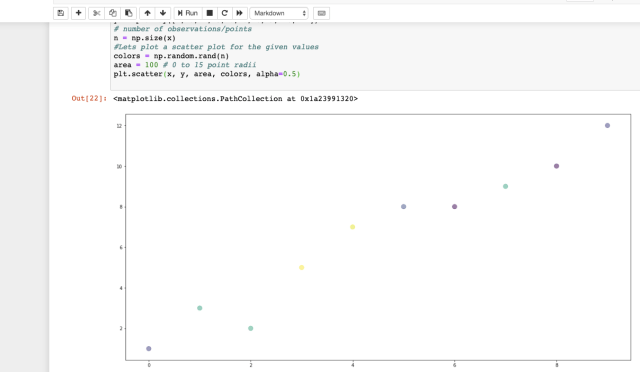最合适的生产线： (Best Fit Line :)
Now we need to find the line which fits best in the above scatter plot so that we can predict the response for any new feature values. (i.e a value of x not present in the dataset). This line is called the regression line. 现在我们需要找到最适合上述散点图的线，以便我们可以预测任何新特征值的响应。 (即数据集中不存在的x值)。 这条线称为回归线 。
The equation of the regression line is represented as: 回归线的方程表示为：
y = bo + b1X +∈ (y= bo + b1X + ∈)
这里， (Here,)
y represents the predicted response value for ith observation. y代表第i次观察的预测响应值 。 b0 and b1 are regression coefficients and represent y-intercept and slope of the regression line respectively. b0和b1是回归系数，分别代表y截距和回归线的斜率 。 ∈, is the residual error. ∈，是残差。 To build our simple linear regression model, we need to learn or estimate the values of regression coefficients b0 and b1. These coefficients will be used to build the model to predict responses. 要构建简单的线性回归模型，我们需要学习或估计回归系数b0和b1的值。 这些系数将用于构建模型以预测响应。
We will make use of the Least Squares technique to find the best fit line. 我们将利用最小二乘技术找到最佳拟合线。
Least squares is a statistical method used to determine the best fit line or the regression line by minimizing the sum of squares created by a mathematical function. The “square” here refers to squaring the distance between a data point and the regression line. The line with the minimum value of the sum of square is the best-fit regression line. 最小二乘法是一种统计方法，用于通过最小化由数学函数创建的平方和来确定最佳拟合线或回归线。 这里的“正方形”是指对数据点和回归线之间的距离进行平方。 平方和最小值最小的线是最佳拟合回归线。  步骤2：计算坡度和截距 (Step2: Calculating slope & intercept)
Let’s do some required calculation in python notebook to fins b0, b1. But before that, it is important to understand a few more formulas which we will perform in the python 让我们在python笔记本中进行一些必要的计算以查找b0，b1。 但在此之前，重要的是要了解我们将在python中执行的更多公式
#bo is intercept#b1 is slopeb0= (Σy)(Σx2) - (Σx)(Σxy)/ n(Σx2) - (Σx)2b1=(slope)= n (Σxy) - (Σx)(Σy) /n(Σx2) - (Σx)2Execute the below-given code in your Jupyter notebook to continue, 在Jupyter笔记本中执行下面给出的代码以继续，
#Step2 calculating slope & intercept #b0= (Σy)(Σx2) - (Σx)(Σxy)/ n(Σx2) - (Σx)2#b1=(slope)= n (Σxy) - (Σx)(Σy) /n(Σx2) - (Σx)2#mean of x and y vector m_x, m_y = np.mean(x), np.mean(y)# calculating cross-deviation and deviation about x SS_xy = np.sum(y*x) - n*m_y*m_x SS_xx = np.sum(x*x) - n*m_x*m_x # calculating regression coefficients b1 = SS_xy / SS_xx b0 = m_y - b1*m_x print("Coefficient b1 is: ",b1 )print("Coefficient b0 is: ",b0 )Run this code and you will see the output as given below: 运行此代码，您将看到如下输出：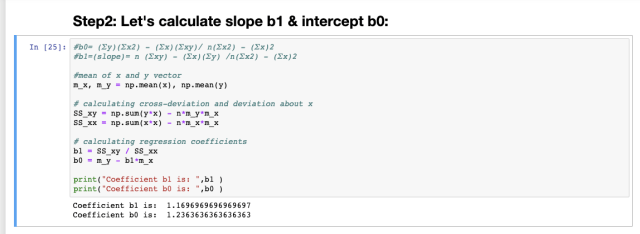So we have the required coefficient, b0= 1.23, b1= 1.16 因此我们有所需的系数b0 = 1.23，b1 = 1.16
第三步：绘制回归线： (Step3 : Plotting the line of regression:)
#Step 3 : Let's plot the scatter plot along with predicted y value #based on our slope & intercept#plotting the actual points as scatter plot plt.scatter(x, y, color = "m", marker = "o", s = 100) # predicted response vector y_pred = b0 + b1*x# plotting the regression line plt.plot(x, y_pred, color = "g") # putting labels plt.xlabel('x') plt.ylabel('y') #show plot plt.show()Execute the above code and run, you will see the output as given below in fig:4, 执行上面的代码并运行，您将看到如图4所示的输出，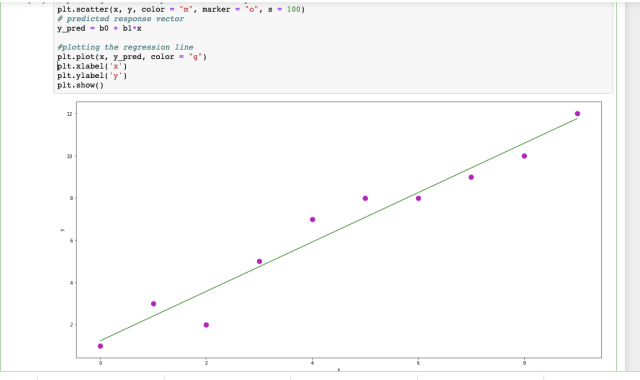步骤5：评估模型：使用R平方 (Step 5: Evaluating the model: Using R-Squared)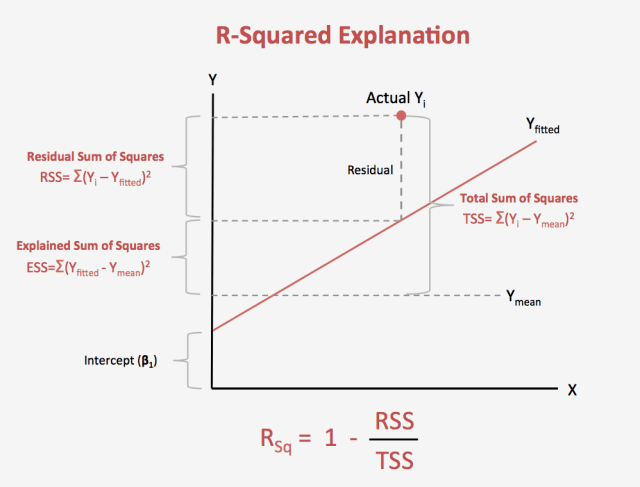Once we have the simple linear regression line( model ) we need to evaluate the same to measure its fitness. We will evaluate the overall fit of a linear model, using the R-squared value 一旦有了简单的线性回归线(模型)，我们就需要对其进行评估以衡量其适用性。 我们将使用R平方值评估线性模型的整体拟合
R平方： (R-Squared:)
R-squared is the proportion of variance explained R平方是解释的方差比例 It is the proportion of variance in the observed data that is explained by the model or the reduction in error over the null model 由模型解释的是观察数据中方差的比例，或者与原始模型相比误差的减少 The null model just predicts the mean of the observed response, and thus it has an intercept and no slope 零模型只是预测观察到的响应的平均值，因此具有截距且没有斜率 R-squared is between 0 and 1 R平方介于0和1之间 Higher values are better because it means that more variance is explained by the model. 值越高越好，因为这意味着模型可以解释更多的方差。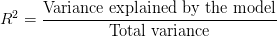使用Python计算R平方： (Calculating R-Squared Using Python:)
水平Y.mean()线 (Horizontal Y.mean() Line)
Next, we will place another line on our data. This is a key step in calculating our r-squared, as you will see in a minute. Write the below-given code and compile it 接下来，我们将在数据上放置另一行。 您将在稍后看到，这是计算r平方的关键步骤。 编写下面给出的代码并进行编译
#plot a horizontal line along mean of yline2 = np.full([m-x],[m_y])plt.scatter(x,y)plt.plot(x,line2, c = 'r')plt.show()The output is given below in fig:5 输出如下图：5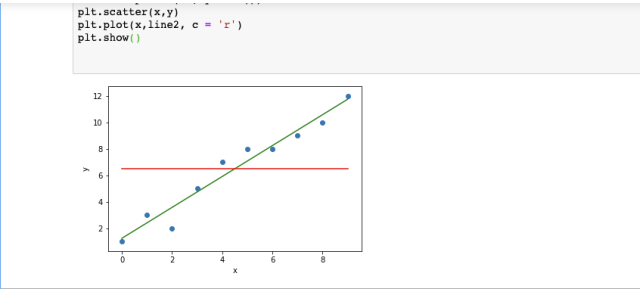Write the below given code and compile to get the r-squared value, 编写以下给出的代码并进行编译以获取r平方值，
differences_line1 = y_pred-yline1sum = 0for i in differences_line1: line1sum = line1sum + (i*i)line1sumprint(line1sum)differences_line2 = line2 — yline2sum = 0for i in differences_line2: line2sum = line2sum + (i*i)line2sumprint(line2sum)#Variance of our linear model: 5.624#Total variance of the target variable: 118.5diff = line2sum-line1sumprint(diff)rsquared= diff/line2sumprint(“R-Squared is : “, rsquared)#Let’s Verify The r-squared we calculated by using sklearn “r2_score” function:from sklearn.metrics import r2_scorer2Score = r2_score(y, y_pred)print(“Rsquared usinf sklearn: “, r2Score)#Observationprint("\nAs r-sqaured value is almost close to 1 , we can easily say that our linear regression model, y_pred = b0 + b1*x is a good fit linear regression line.")R-Squared value comes out to be: 0.95 R平方值得出为 ：0.95
测量R平方： (Measuring R-Squared:)
Higher values are better because it means that more variance is explained by the model. 值越高越好，因为这意味着模型可以解释更多的方差。
观察： (Observation:)
In our case, the r-squared value has come quite high to almost 0.95 very close to 1. So we can say that our model is better as it explains a larger variance in the data. 在我们的例子中，r平方值非常高，非常接近1，几乎达到0.95。因此，可以说我们的模型更好，因为它解释了数据中的较大差异。
R平方限制： (R-squared Limitations:)
You cannot use R-squared to determine whether the coefficient estimates and predictions are biased, which is why you must assess the residual plots. 您不能使用R平方来确定系数估计和预测是否有偏差，这就是为什么您必须评估残差图的原因。
Caution: R-squared does not indicate if a regression model provides an adequate fit to your data. A good model can have a low R2 value. On the other hand, a biased model can have a high R2 value! 注意 ：R平方不会指示回归模型是否可以完全适合您的数据。 好的模型可能具有较低的R2值。 另一方面，有偏差的模型可以具有较高的R2值！
So there is one another type of R2: adjusted R-squared and predicted R-squared. These two statistics address particular problems with R-squared. They provide extra information by which you can assess your regression model’s goodness-of-fit. We will cover this later. 因此，还有另一种R2：调整后的R平方和预测的R平方。 这两个统计数据解决了R平方的特定问题。 它们提供了额外的信息，您可以通过这些信息评估回归模型的拟合优度。 我们将在后面介绍。
Let’s understand the same example: 让我们理解相同的示例：
使用Sklearn Python库： (Using Sklearn Python Library:)
Here we can perform the same calculation to find the simple linear regression model using sklearn in just a few lines: 在这里，我们可以执行相同的计算，以使用sklearn在几行中找到简单的线性回归模型：
Here we go, 开始了，
import numpy as npimport pandas as pdimport matplotlib.pyplot as pltimport seaborn as snsfrom sklearn.linear_model import LinearRegressionx = np.array([0, 1, 2, 3, 4, 5, 6, 7, 8, 9]).reshape(-1,1) y = np.array([1, 3, 2, 5, 7, 8, 8, 9, 10, 12]) #invoke the LinearRegression function and find the bestfit model on #our given dataregression_model = LinearRegression()regression_model.fit(x, y) #this will give the best fit line # Let us explore the coefficients for each of the independent #attributesb1 = regression_model.coef_b0 = regression_model.intercept_print("b1 is: {} and b0 is: {}".format(b1, b0))plt.scatter(x, y, color = "m", marker = "o", s = 100) plt.plot(x, b1*x+b0)When you write & compile the above-given code snippet you will get the scatter plot with a line of regression, as shown below: 在编写和编译上述代码片段时，您将获得带有一排回归线的散点图，如下所示：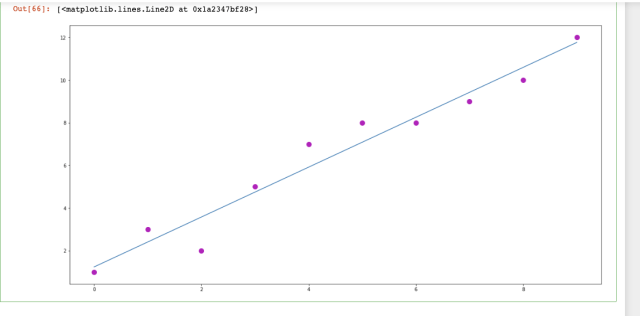让我们计算R2分数： (Let’s Calculate R2 Score:)
#sklearn has a function to calculate R-Squared value as seen #belowfrom sklearn.metrics import r2_score#y_pred is the predicted value which our linear regression model #predicted when we plotted the best fit line y_pred= regression_model.predict(x)r2Score = r2_score(y, y_pred) #here y is our original value print(r2Score)Output: 输出：
When you will compile the above code you will get the R-squared value to be 0.95 which we also calculated mathematically previously. 当您编译以上代码时，您将获得R平方值为0.95，这也是我们先前通过数学计算得出的。
加起来： (Summing Up:)
We covered the basics of simple linear regression and understood how we can find the linear regression model with one predictor value X. But there is more to the linear regression. We will not be often dealing with only one predictor value instead we will have large data sets with multiple independent values where you need to deal with multiple linear regression & polynomial type linear regression 我们介绍了简单线性回归的基础知识，并了解了如何找到具有一个预测值X的线性回归模型。但是线性回归还有很多。 我们将不会经常只处理一个预测值，而是会拥有包含多个独立值的大型数据集，您需要处理多个线性回归和多项式线性回归
下一步是什么？ (What’s Next?)
Will cover the following topics in the next part of Linear Regression, 在线性回归的下一部分中将涵盖以下主题，
Multiple linear regression model with one case study 多元线性回归模型与一个案例研究 polynomial regression model 多项式回归模型 Concept of underfitting & overfitting 欠拟合与过拟合的概念 Various techniques of error minimization in linear regression with examples 线性回归中的各种误差最小化技术与示例 Linear regression learning models like gradient descent, OLS, Regularization 线性回归学习模型，例如梯度下降 ， OLS ， 正则化 Thanks for being with me all along, will be back soon, keep loving keep sharing. 感谢您一直以来与我在一起，很快就会回来，继续爱着，继续分享。
翻译自: https://medium.com/swlh/linear-regression-explained-for-beginners-in-machine-learning-9e74f168d8a8机器学习线性回归学习心得
展开全文python 人工智能 深度学习
• 对于练习题的详细内容，和课程中推荐的octave编程实现，请见：吴恩达机器学习线性回归练习题：多变量线性回归（octave实现） 这边只贴出整个多变量线性回归步骤的Python实现代码，每个步骤在代码中有注释说明，变量...
练习题背景：网易云课堂->吴恩达机器学习课程->线性回归练习题->多变量线性回归

对于练习题的详细内容，和课程中推荐的octave编程实现，请见：吴恩达机器学习线性回归练习题：多变量线性回归（octave实现）

这边只贴出整个多变量线性回归步骤的Python实现代码，每个步骤在代码中有注释说明，变量和函数的命名也基本与练习题资料一致。

全部代码：

import pandas
import numpy
import matplotlib.pyplot as plt

def featureNormalization(X):
"""
数据标准化
:param X:
:return:
"""
mu = numpy.mean(X, axis=0)
# ddof的设置，会改变标准差的结算结果，因为总体误差和样本误差的计算公式不一样
sigma = numpy.std(X, axis=0, ddof=1)

X_norm = (X - mu) / sigma

return X_norm, mu, sigma

def computeCostMulti(X, y, theta):
"""
计算损失函数
:param X:
:param y:
:param theta:
:return:
"""
m = X.shape
costs = X.dot(theta) - y
total_cost = costs.transpose().dot(costs) / (2 * m)

def gradientDescentMulti(X, y, theta, alpha, iterNum):
"""
梯度下降实现
:param X:
:param y:
:param theta:
:param alpha:
:param iterNum:
:return:
"""
m = len(X)

J_history = list()

for i in range(0, iterNum):
costs = X.dot(theta) - y
theta = theta - numpy.transpose(costs.transpose().dot(X) * (alpha / m))

J_history.append(computeCostMulti(X, y, theta))

return theta, J_history

def learningRatePlot(X_norm, y):
"""
不同学习速率下的梯度下降比较
:param X_norm:
:param y:
:return:
"""
colors = ['b', 'g', 'r', 'c', 'm', 'y', 'k']
plt.figure()
iter_num = 50
# 如果学习速率取到3，损失函数的结果随着迭代次数增加而发散，值越来越大，不太适合在同一幅图中展示
for i, al in enumerate([0.01, 0.03, 0.1, 0.3, 1]):
ta = numpy.zeros((X_norm.shape, 1))
ta, J_history = gradientDescentMulti(X_norm, y, ta, al, iter_num)

plt.plot([i for i in range(len(J_history))], J_history, colors[i], label=str(al))

plt.title("learning rate")
plt.legend()
plt.show()

def normalEquation(X, y):
"""
正规方程实现
:param X:
:param y:
:return:
"""
return numpy.linalg.inv(X.transpose().dot(X)).dot(X.transpose()).dot(y)

if __name__ == '__main__':
# 读取数据
data_path = r'D:\ML\AndrewNg\machine-learning-ex1\ex1\ex1data2.txt'

# 切分特征和目标， 注意：索引是从0开始的
X = data.iloc[:, 0:2].values
y = data.iloc[:, 2:3].values

# 数据标准化
X_norm, mu, sigma = featureNormalization(X)

ones = numpy.ones((X_norm.shape, 1))

# 假设函数中考虑截距的情况下，给每个样本增加一个为1的特征
X_norm = numpy.c_[ones, X_norm]

# 初始化theta
theta = numpy.zeros((X_norm.shape, 1))

# 梯度下降学习速率为0.01
alpha = 0.01
# 梯度下降迭代次数为400
iterNum = 400

# 梯度下降
theta, J_history = gradientDescentMulti(X_norm, y, theta, alpha, iterNum)

# 画出梯度下降过程中的收敛情况
plt.figure()
plt.plot([i for i in range(len(J_history))], J_history)
plt.title("learning rate: %f" % alpha)
plt.show()

# 使用不同学习速率下的收敛情况
learningRatePlot(X_norm, y)

# 预测面积为1650，卧室数量为3的房子价格
x_pre = numpy.array([1650, 3])

x_pre_norm = (x_pre - mu) / sigma
numpy_ones = numpy.ones((1,))
x_pre_norm = numpy.concatenate((numpy.ones((1,)), x_pre_norm))
price = x_pre_norm.dot(theta)
print("通过梯度下降求解的参数预测面积1650、卧室数量3的房子价格为：%f" % price)

# 下面使用正规方程计算theta
X_ = numpy.c_[ones, data.iloc[:, 0:2].values]
y_ = data.iloc[:, 2:3].values

theta = normalEquation(X_, y)

# 预测面积为1650，卧室数量为3的房子价格
x_pre = numpy.array([1, 1650, 3])
price = x_pre.dot(theta)
print("通过正规方程求解的参数预测面积1650、卧室数量3的房子价格为：%f" % price)

代码执行结果：

通过梯度下降求解的参数预测面积1650、卧室数量3的房子价格为：289314.620338
通过正规方程求解的参数预测面积1650、卧室数量3的房子价格为：293081.464335

代码运行过程中会生成两张图：

迭代次数为400，学习速率为0.01时，梯度下降的收敛情况：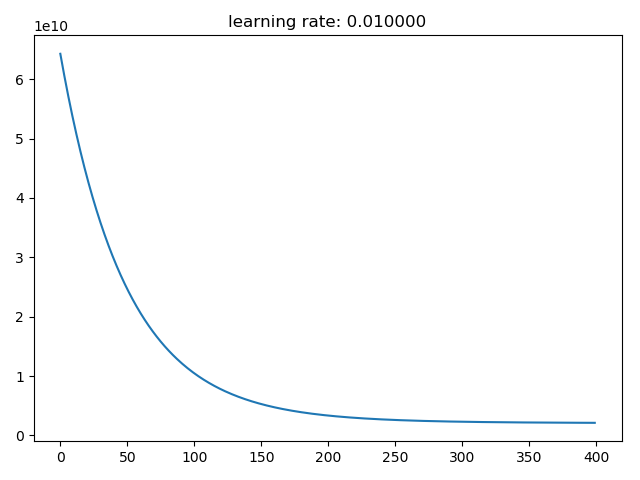迭代次数为50时，不同学习速率下梯度下降的收敛情况：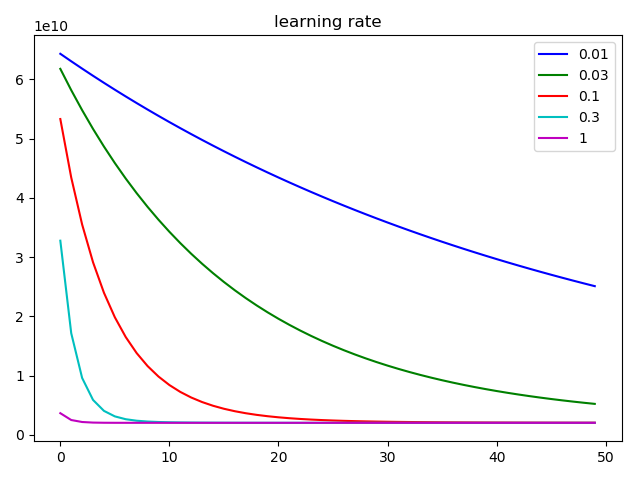展开全文吴恩达 编程题
• 线性回归机器学习最基础的，也是最经典的算法，下面就来介绍这个算法。 假如我们要去银行贷款，那么银行会贷给我们多少钱，我们可以通过特征来计算出来。 数据：工资和年龄（2个特征） 目标：预测银行会贷款...
• 回归是一种解题的方法，或者说是“学习的方法”，也是机器学习中比较重要的概念。 回归一词的英文是Regression,单词原型regress大概的意思是“回退，倒退，退化”。 其实Regression回归分析的意思借用了这么一层含义...人工智能 python
• 单变量线性回归 绘制散点图 相关系数R 拆分训练集和测试集 多变量线性回归 数据检验（判断是否可以做线性回归） 训练线性回归模型 先甩几个典型的线性回归的模型，帮助大家捡起那些年被忘记的数学。 ● 单...python
• 机器学习常用来解决相关分析和回归分析的问题，有时候大家会混淆两者之间的差异，这里通过对比分析来说明两者的区别和联系，最后会以调用sklearn包中LinearRegression方法进行简单线性回归分析为例，说明如何使用...Python
• 线性回归的损失函数 假设以波士顿房价为例子，真实的数据之间存在这样的关系 真实关系：真实房子价格 = 0.02×中心区域的距离 + 0.04×城市一氧化氮浓度 + (-0.12×自住房平均房价) + 0.254×城镇犯罪率 那么现在呢...python 深度学习 人工智能
• 文章目录线性回归学习目标2.1 线性回归简介1 线性回归应用场景2 什么是线性回归2.1 定义与公式2.2 线性回归的特征与目标的关系分析 学习目标 掌握线性回归的实现过程 应用LinearRegression或SGDRegressor实现回归...人工智能 python 深度学习 算法
• 本文介绍机器学习中的线性模型的基本形式和求解线性回归方程方法 设问题为：判断一个同学对机器学习算法的掌握程度 有以下观点：1.数学基础 2.高级语言掌握程度 ...
• 本文致力于手把手教你实现一个最简单的机器学习模型--一元线性回归模型。短短的14行代码，就实现了。希望读完以后，你也能自己实现它。并对线性回归有更好的了解，或者从不了解到了解。Python 算法
• 线性回归(Linear regressions)和逻辑回归(Logistic regressions)是人们学习算法的第一个预测模型。因此它们很常见，还有许多分析人员认为它们是仅有的回归模型，部分分析师认为它们是所有回归模型中最重要的。 事实...
• 听闻有个同事每天买彩票，想到机器学习用来预测彩票也是不错的。 于是今天尝试了下（事先声明，最后测试发现一点也不准，所以。。。别想太多了。。哈哈） 具体代码如下： 首先我在某彩票网站上找到了大量双色球的...
• 机器学习监督学习算法分为分类算法和回归算法两种，其实就是根据类别标签分布类型为离散型、连续性而定义的。回归算法用于连续型分布预测，针对的是数值型的样本，使用回归，可以在给定输入的时候预测出一个数值，这...人工智能 回归算法
• 线性回归线性回归找到最佳拟合直线回归的目的是预测数值型数据，根据输入写出一个目标值的计算公式，这个公式就是回归方程（regression equation），变量前的系数（比如一元一次方程）称为回归系数（regression ...
• ## 机器学习-线性回归总结

万次阅读 多人点赞 2019-01-19 20:38:20
最近学习了线性回归的模型，也是机器学习中最基础的一种模型。在此，总结一下线性回归的模型介绍、梯度下降以及正规方程。 回归问题 线性回归，顾名思义，属于回归问题。既然是回归问题，那必然属于监督学习。 在...
•算法...